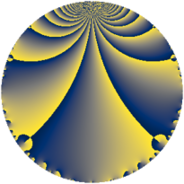# Properties

 Label 5586.2.a.boLevel $5586$ Weight $2$ Character orbit 5586.a Self dual yes Analytic conductor $44.604$ Analytic rank $1$ Dimension $2$ CM no Inner twists $1$

# Related objects

## Newspace parameters

 Level: $$N$$ $$=$$ $$5586 = 2 \cdot 3 \cdot 7^{2} \cdot 19$$ Weight: $$k$$ $$=$$ $$2$$ Character orbit: $$[\chi]$$ $$=$$ 5586.a (trivial)

## Newform invariants

 Self dual: yes Analytic conductor: $$44.6044345691$$ Analytic rank: $$1$$ Dimension: $$2$$ Coefficient field: $$\Q(\sqrt{2})$$ Defining polynomial: $$x^{2} - 2$$ Coefficient ring: $$\Z[a_1, \ldots, a_{11}]$$ Coefficient ring index: $$1$$ Twist minimal: yes Fricke sign: $$1$$ Sato-Tate group: $\mathrm{SU}(2)$

## $q$-expansion

Coefficients of the $$q$$-expansion are expressed in terms of $$\beta = \sqrt{2}$$. We also show the integral $$q$$-expansion of the trace form.

 $$f(q)$$ $$=$$ $$q + q^{2} + q^{3} + q^{4} + q^{6} + q^{8} + q^{9} +O(q^{10})$$ $$q + q^{2} + q^{3} + q^{4} + q^{6} + q^{8} + q^{9} + ( -4 + \beta ) q^{11} + q^{12} -2 \beta q^{13} + q^{16} + ( -4 + 2 \beta ) q^{17} + q^{18} - q^{19} + ( -4 + \beta ) q^{22} -3 \beta q^{23} + q^{24} -5 q^{25} -2 \beta q^{26} + q^{27} -8 q^{29} + ( -2 + 4 \beta ) q^{31} + q^{32} + ( -4 + \beta ) q^{33} + ( -4 + 2 \beta ) q^{34} + q^{36} -2 q^{37} - q^{38} -2 \beta q^{39} + ( -4 + \beta ) q^{41} + ( -4 - 2 \beta ) q^{43} + ( -4 + \beta ) q^{44} -3 \beta q^{46} + ( -2 - 4 \beta ) q^{47} + q^{48} -5 q^{50} + ( -4 + 2 \beta ) q^{51} -2 \beta q^{52} -2 q^{53} + q^{54} - q^{57} -8 q^{58} + ( 4 - \beta ) q^{61} + ( -2 + 4 \beta ) q^{62} + q^{64} + ( -4 + \beta ) q^{66} -3 \beta q^{67} + ( -4 + 2 \beta ) q^{68} -3 \beta q^{69} + 10 \beta q^{71} + q^{72} + ( 8 + \beta ) q^{73} -2 q^{74} -5 q^{75} - q^{76} -2 \beta q^{78} + ( -4 + 7 \beta ) q^{79} + q^{81} + ( -4 + \beta ) q^{82} + ( -4 - 4 \beta ) q^{83} + ( -4 - 2 \beta ) q^{86} -8 q^{87} + ( -4 + \beta ) q^{88} + ( 4 - \beta ) q^{89} -3 \beta q^{92} + ( -2 + 4 \beta ) q^{93} + ( -2 - 4 \beta ) q^{94} + q^{96} + ( 12 + 2 \beta ) q^{97} + ( -4 + \beta ) q^{99} +O(q^{100})$$ $$\operatorname{Tr}(f)(q)$$ $$=$$ $$2q + 2q^{2} + 2q^{3} + 2q^{4} + 2q^{6} + 2q^{8} + 2q^{9} + O(q^{10})$$ $$2q + 2q^{2} + 2q^{3} + 2q^{4} + 2q^{6} + 2q^{8} + 2q^{9} - 8q^{11} + 2q^{12} + 2q^{16} - 8q^{17} + 2q^{18} - 2q^{19} - 8q^{22} + 2q^{24} - 10q^{25} + 2q^{27} - 16q^{29} - 4q^{31} + 2q^{32} - 8q^{33} - 8q^{34} + 2q^{36} - 4q^{37} - 2q^{38} - 8q^{41} - 8q^{43} - 8q^{44} - 4q^{47} + 2q^{48} - 10q^{50} - 8q^{51} - 4q^{53} + 2q^{54} - 2q^{57} - 16q^{58} + 8q^{61} - 4q^{62} + 2q^{64} - 8q^{66} - 8q^{68} + 2q^{72} + 16q^{73} - 4q^{74} - 10q^{75} - 2q^{76} - 8q^{79} + 2q^{81} - 8q^{82} - 8q^{83} - 8q^{86} - 16q^{87} - 8q^{88} + 8q^{89} - 4q^{93} - 4q^{94} + 2q^{96} + 24q^{97} - 8q^{99} + O(q^{100})$$

## Embeddings

For each embedding $$\iota_m$$ of the coefficient field, the values $$\iota_m(a_n)$$ are shown below.

For more information on an embedded modular form you can click on its label.

Label $$\iota_m(\nu)$$ $$a_{2}$$ $$a_{3}$$ $$a_{4}$$ $$a_{5}$$ $$a_{6}$$ $$a_{7}$$ $$a_{8}$$ $$a_{9}$$ $$a_{10}$$
1.1
 −1.41421 1.41421
1.00000 1.00000 1.00000 0 1.00000 0 1.00000 1.00000 0
1.2 1.00000 1.00000 1.00000 0 1.00000 0 1.00000 1.00000 0
 $$n$$: e.g. 2-40 or 990-1000 Significant digits: Format: Complex embeddings Normalized embeddings Satake parameters Satake angles

## Atkin-Lehner signs

$$p$$ Sign
$$2$$ $$-1$$
$$3$$ $$-1$$
$$7$$ $$1$$
$$19$$ $$1$$

## Inner twists

This newform does not admit any (nontrivial) inner twists.

## Twists

By twisting character orbit
Char Parity Ord Mult Type Twist Min Dim
1.a even 1 1 trivial 5586.2.a.bo yes 2
7.b odd 2 1 5586.2.a.bj 2

By twisted newform orbit
Twist Min Dim Char Parity Ord Mult Type
5586.2.a.bj 2 7.b odd 2 1
5586.2.a.bo yes 2 1.a even 1 1 trivial

## Hecke kernels

This newform subspace can be constructed as the intersection of the kernels of the following linear operators acting on $$S_{2}^{\mathrm{new}}(\Gamma_0(5586))$$:

 $$T_{5}$$ $$T_{11}^{2} + 8 T_{11} + 14$$ $$T_{13}^{2} - 8$$ $$T_{17}^{2} + 8 T_{17} + 8$$

## Hecke characteristic polynomials

$p$ $F_p(T)$
$2$ $$( -1 + T )^{2}$$
$3$ $$( -1 + T )^{2}$$
$5$ $$T^{2}$$
$7$ $$T^{2}$$
$11$ $$14 + 8 T + T^{2}$$
$13$ $$-8 + T^{2}$$
$17$ $$8 + 8 T + T^{2}$$
$19$ $$( 1 + T )^{2}$$
$23$ $$-18 + T^{2}$$
$29$ $$( 8 + T )^{2}$$
$31$ $$-28 + 4 T + T^{2}$$
$37$ $$( 2 + T )^{2}$$
$41$ $$14 + 8 T + T^{2}$$
$43$ $$8 + 8 T + T^{2}$$
$47$ $$-28 + 4 T + T^{2}$$
$53$ $$( 2 + T )^{2}$$
$59$ $$T^{2}$$
$61$ $$14 - 8 T + T^{2}$$
$67$ $$-18 + T^{2}$$
$71$ $$-200 + T^{2}$$
$73$ $$62 - 16 T + T^{2}$$
$79$ $$-82 + 8 T + T^{2}$$
$83$ $$-16 + 8 T + T^{2}$$
$89$ $$14 - 8 T + T^{2}$$
$97$ $$136 - 24 T + T^{2}$$